# RD Sharma Solutions for Class 8 Math Chapter 6 - Algebraic Expressions and Identities (Part-2) Notes | Study RD Sharma Solutions for Class 8 Mathematics - Class 8

## Class 8: RD Sharma Solutions for Class 8 Math Chapter 6 - Algebraic Expressions and Identities (Part-2) Notes | Study RD Sharma Solutions for Class 8 Mathematics - Class 8

The document RD Sharma Solutions for Class 8 Math Chapter 6 - Algebraic Expressions and Identities (Part-2) Notes | Study RD Sharma Solutions for Class 8 Mathematics - Class 8 is a part of the Class 8 Course RD Sharma Solutions for Class 8 Mathematics.
All you need of Class 8 at this link: Class 8

#### Question 4: Find each of the following product: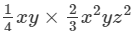#### Answer 4: To multiply algebraic expressions, we use commutative and associative laws along with the the law of indices, that is, am×an=am+nam×an=am+n.

We have: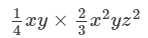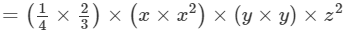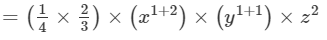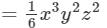Thus, the answer is 1/6 x3y2z216x3y2z2.

#### Question 5: Find each of the following product: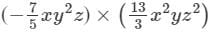#### Answer 5: To multiply algebraic expressions, we use commutative and associative laws along with the law of indices, i.e., am×an=am+nam×an=am+n.

We have: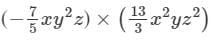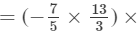(x×x2)×(y2×y)×(z×z2)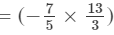×(x1+2)×(y2+1)×(z1+2)

= - 91/15 x3y3x3

Thus, the answer is = - 91/15 x3y3x3

#### Question 6: Find each of the following product: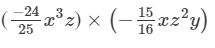#### Answer 6: To multiply algebraic expressions, we use commutative and associative laws along with the law of indices, i.e., am×an=am+nam×an=am+n.

We have: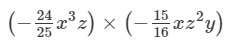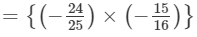×(x3×x)×(z×z2)×y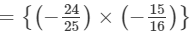×(x3+1)×(z1+2)×y

9/10 x4yz3=910x4yz3

Thus, the answer is  9/10 x4yz3=910x4yz3

#### Question 7: Find each of the following product: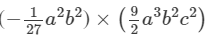####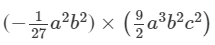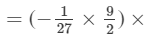(a2×a3)×(b2×b2)×c2(a2+3)×(b2+2)×c2

= - 1/6 a5b4c2

Thus, the answer is = - 1/6 a5b4c2

#### Answer 8: To multiply algebraic expressions, we use commutative and associative laws along with the law of indices, i.e., am×an=am+nam×an=am+n.We have: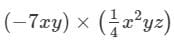=(7×1/4)×(x×x2)×(y×y)×z

=(7×1/4)×(x1+2)×(y1+1)×z

= 7/4 x3y2z

Thus, the answer is  7/4 x3y2z

#### Question 12: Find each of the following product: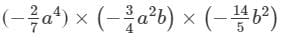#### Answer 12: To multiply algebraic expressions, we use commutative and associative laws along with the law of indices, i.e., am×an=am+nam×an=am+n.We have: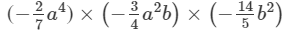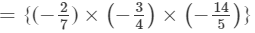×(a4×a2)×(b×b2)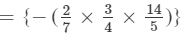×a4+2×b1+2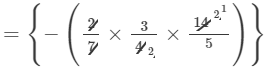×a6×b3

=− 3/5 a6b3

Thus, the answer is  − 3/5 a6b3

#### Question 13: Find each of the following product: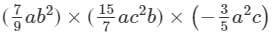#### Answer 13: To multiply algebraic expressions, we use commutative and associative laws along with the law of indices, i.e., am×an=am+nam×an=am+n.We have: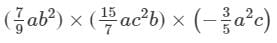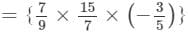×(a×a×a2)×(b2×b)×(c2×c)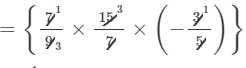×(a×a×a2)×(b2×b)×(c2×c)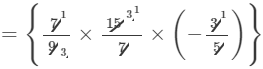×(a1+1+2)×(b2+1)×(c2+1)

=a4b3c3

#### Question 14: Find each of the following product: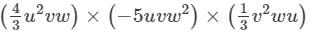#### (4/3 u2vw)×(−5uvw2)×(1/3 v2wu)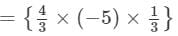×(u2×u×u)×(v×v×v2)×(w×w2×w)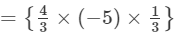×(u2+1+1)×(v1+1+2)×(w1+2+1)

= 20/9 u4v4w4

Thus, the answer is  20/9 u4v4w4

#### Answer 15: To multiply algebraic expressions, we use commutative and associative laws along with the law of indices, i.e., am×an=am+nam×an=am+n.We have:

(0.5x)×(1/3 xy2z4)×(24x2yz)

=(0.5×1/3×24)×(x×x×x2)×(y2×y)×(z4×z)

=(0.5×1/3×24)×(x1+1+2)×(y2+1)×(z4+1)

=4x4y3z5

#### Question 16: Find each of the following product:

(4/3 pq2)×(1/4 p2r)×(16p2q2r2)43pq2×-14p2r×16p2q2r2

#### Answer 16: To multiply algebraic expressions, we use commutative and associative laws along with the law of indices, i.e., am×an=am+nam×an=am+n.We have:

(4/3 pq2)×(1/4 p2r)×(16p2q2r2)

={4/3×(−1/4)×16}×(p×p2×p2)×(q2×q2)×(r×r2)

={4/3×(− 1/4)×16}×(p1+2+2)×(q2+2)×(r1+2)

= 16/3p5q4r3

Thus, the answer is 1/3 p5q4r3-13p5q4r3.

#### Answer 19: We have to find the product of the expression in order to express it as a monomial.To multiply algebraic expressions, we use commutative and associative laws along with the law of indices, i.e., am×an=am+nam×an=am+n.

We  have:

(4x2)×(3x)×(4/5 x3)

={4×(3)×4/5}×(x2×x×x3)

={4×(3)×4/5}×(x2+1+3)

=− 48/5x6

(4x2)×(3x)×(4/5 x3)=− 48/5x6

Substituting x = 1 in LHS, we get:

LHS=(4x2)×(3x)×(4/5 x3)

=(4×12)×(3×1)×(4/5 ×13)

=4×(3)×4/5

=48/5

Putting x = 1 in RHS, we get:

RHS= 48/5 x6
=48/5×16

=48/5

LHS = RHS for = 1; therefore, the result is correct
Thus, the answer is − 48/5 x6-485x6.

#### Answer 20: We have to find the product of the expression in order to express it as a monomial.To multiply algebraic expressions, we use commutative and associative laws along with the laws of indices, i.e., am×an=am+n and (am)n=amn am×an=am+n and amn=amn .We have:(5x4)×(x2)3×(2x)2 =(5x4)×(x6)×(22×x2)=(5×22)×(x4×x6×x2)=(5×22)×(x4+6+2)=20x12∴ (5x4)×(x2)3×(2x)2 =20x12

Substituting x = 1 in LHS, we get:
LHS=(5x4)×(x2)3×(2